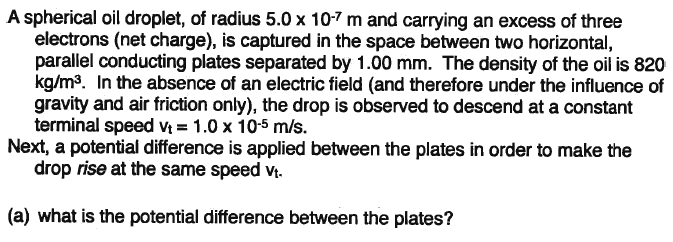Parallel Plate Capacitor

Homework StatementThe Attempt at a Solution

All I need to know is the acceleration acting on the particle after the potential is applied.

$v_f^2 = v_i^2 + 2ad$
$(1*10^{-5})^2 = -(1*10^{-5})^2 + 2a(1*10^{-3})$
$a = 1*10^{-7}$ m/s2

Is this the correct approach to finding acceleration?

I don't think so because your acceleration is actually not going to me constant. Recall that the equation you used only applies for a constant acceleration.

I think you should go along the lines of qE = ma to find this.

You're given enough info to find the electric field between the plates, you are given the net charge of the droplet, and density multiplied by volume will give you the mass of your droplet.

How do I find the electric field between the plates? I don't have the charge of the plates.

gneill
Mentor
Draw a free body diagram for the oil droplet for both of the given cases. What forces are acting? Can you draw any conclusions about the magnitude of the force due to air friction when the droplet is moving at speed vt?

By drawing a FBD for the first case, I was able to determine the force due to air friction is equal to force due to gravity. For the second FBD, I was able to determine that the force due to the electric field is equal to the sum of the forces due to air friction and gravity. Therefore, the force due to the electric field is equal to twice the force due to gravity. Is this correct?

gneill
Mentor
By drawing a FBD for the first case, I was able to determine the force due to air friction is equal to force due to gravity. For the second FBD, I was able to determine that the force due to the electric field is equal to the sum of the forces due to air friction and gravity. Therefore, the force due to the electric field is equal to twice the force due to gravity. Is this correct?

Sounds good!

What does that tell you about the magnitude of the electric field between the plates (when the plates are given a potential difference)? Can you write an expression?

•1 person
$E = \frac{2mg}{q}$

Thanks for the help.